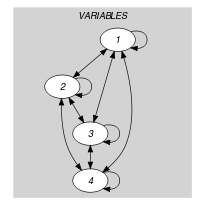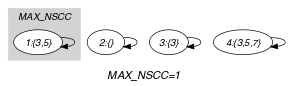## 5.13. alldifferent_between_sets

Origin

ILOG

Constraint

$\mathrm{𝚊𝚕𝚕𝚍𝚒𝚏𝚏𝚎𝚛𝚎𝚗𝚝}_\mathrm{𝚋𝚎𝚝𝚠𝚎𝚎𝚗}_\mathrm{𝚜𝚎𝚝𝚜}\left(\mathrm{𝚅𝙰𝚁𝙸𝙰𝙱𝙻𝙴𝚂}\right)$

Synonyms

$\mathrm{𝚊𝚕𝚕}_\mathrm{𝚗𝚞𝚕𝚕}_\mathrm{𝚒𝚗𝚝𝚎𝚛𝚜𝚎𝚌𝚝}$, $\mathrm{𝚊𝚕𝚕𝚍𝚒𝚏𝚏}_\mathrm{𝚋𝚎𝚝𝚠𝚎𝚎𝚗}_\mathrm{𝚜𝚎𝚝𝚜}$, $\mathrm{𝚊𝚕𝚕𝚍𝚒𝚜𝚝𝚒𝚗𝚌𝚝}_\mathrm{𝚋𝚎𝚝𝚠𝚎𝚎𝚗}_\mathrm{𝚜𝚎𝚝𝚜}$, $\mathrm{𝚊𝚕𝚕𝚍𝚒𝚏𝚏}_\mathrm{𝚘𝚗}_\mathrm{𝚜𝚎𝚝𝚜}$, $\mathrm{𝚊𝚕𝚕𝚍𝚒𝚜𝚝𝚒𝚗𝚌𝚝}_\mathrm{𝚘𝚗}_\mathrm{𝚜𝚎𝚝𝚜}$, $\mathrm{𝚊𝚕𝚕𝚍𝚒𝚏𝚏𝚎𝚛𝚎𝚗𝚝}_\mathrm{𝚘𝚗}_\mathrm{𝚜𝚎𝚝𝚜}$.

Argument
 $\mathrm{𝚅𝙰𝚁𝙸𝙰𝙱𝙻𝙴𝚂}$ $\mathrm{𝚌𝚘𝚕𝚕𝚎𝚌𝚝𝚒𝚘𝚗}\left(\mathrm{𝚟𝚊𝚛}-\mathrm{𝚜𝚟𝚊𝚛}\right)$
Restriction
$\mathrm{𝚛𝚎𝚚𝚞𝚒𝚛𝚎𝚍}$$\left(\mathrm{𝚅𝙰𝚁𝙸𝙰𝙱𝙻𝙴𝚂},\mathrm{𝚟𝚊𝚛}\right)$
Purpose

Enforce all sets of the collection $\mathrm{𝚅𝙰𝚁𝙸𝙰𝙱𝙻𝙴𝚂}$ to be distinct.

Example
$\left(〈\mathrm{𝚟𝚊𝚛}-\left\{3,5\right\},\mathrm{𝚟𝚊𝚛}-\varnothing ,\mathrm{𝚟𝚊𝚛}-\left\{3\right\},\mathrm{𝚟𝚊𝚛}-\left\{3,5,7\right\}〉\right)$

The $\mathrm{𝚊𝚕𝚕𝚍𝚒𝚏𝚏𝚎𝚛𝚎𝚗𝚝}_\mathrm{𝚋𝚎𝚝𝚠𝚎𝚎𝚗}_\mathrm{𝚜𝚎𝚝𝚜}$ constraint holds since all the sets $\left\{3,5\right\}$, $\varnothing$, $\left\{3\right\}$ and $\left\{3,5,7\right\}$ are distinct.

Typical
$|\mathrm{𝚅𝙰𝚁𝙸𝙰𝙱𝙻𝙴𝚂}|>2$
Symmetry

Items of $\mathrm{𝚅𝙰𝚁𝙸𝙰𝙱𝙻𝙴𝚂}$ are permutable.

Arg. properties

Contractible wrt. $\mathrm{𝚅𝙰𝚁𝙸𝙰𝙱𝙻𝙴𝚂}$.

Usage

This constraint was available in some configuration library offered by Ilog.

Algorithm

A filtering algorithm for the $\mathrm{𝚊𝚕𝚕𝚍𝚒𝚏𝚏𝚎𝚛𝚎𝚗𝚝}_\mathrm{𝚋𝚎𝚝𝚠𝚎𝚎𝚗}_\mathrm{𝚜𝚎𝚝𝚜}$ is proposed by C.-G. Quimper and T. Walsh in [QuimperWalsh05] and a longer version is available in [QuimperWalshReport05] and in [QuimperWalsh06].

specialisation: $\mathrm{𝚊𝚕𝚕𝚍𝚒𝚏𝚏𝚎𝚛𝚎𝚗𝚝}$ ($\mathrm{𝚜𝚎𝚝}\mathrm{𝚟𝚊𝚛𝚒𝚊𝚋𝚕𝚎}$ replaced by $\mathrm{𝚟𝚊𝚛𝚒𝚊𝚋𝚕𝚎}$).

Keywords
Arc input(s)

$\mathrm{𝚅𝙰𝚁𝙸𝙰𝙱𝙻𝙴𝚂}$

Arc generator
$\mathrm{𝐶𝐿𝐼𝑄𝑈𝐸}$$↦\mathrm{𝚌𝚘𝚕𝚕𝚎𝚌𝚝𝚒𝚘𝚗}\left(\mathrm{𝚟𝚊𝚛𝚒𝚊𝚋𝚕𝚎𝚜}\mathtt{1},\mathrm{𝚟𝚊𝚛𝚒𝚊𝚋𝚕𝚎𝚜}\mathtt{2}\right)$

Arc arity
Arc constraint(s)
$\mathrm{𝚎𝚚}_\mathrm{𝚜𝚎𝚝}$$\left(\mathrm{𝚟𝚊𝚛𝚒𝚊𝚋𝚕𝚎𝚜}\mathtt{1}.\mathrm{𝚟𝚊𝚛},\mathrm{𝚟𝚊𝚛𝚒𝚊𝚋𝚕𝚎𝚜}\mathtt{2}.\mathrm{𝚟𝚊𝚛}\right)$
Graph property(ies)
$\mathrm{𝐌𝐀𝐗}_\mathrm{𝐍𝐒𝐂𝐂}$$\le 1$

Graph class
$\mathrm{𝙾𝙽𝙴}_\mathrm{𝚂𝚄𝙲𝙲}$

Graph model

We generate a clique with binary set equalities constraints between each pair of vertices (including a vertex and itself) and state that the size of the largest strongly connected component should not exceed 1.

Parts (A) and (B) of Figure 5.13.1 respectively show the initial and final graph associated with the Example slot. Since we use the $\mathrm{𝐌𝐀𝐗}_\mathrm{𝐍𝐒𝐂𝐂}$ graph property we show one of the largest strongly connected components of the final graph. The $\mathrm{𝚊𝚕𝚕𝚍𝚒𝚏𝚏𝚎𝚛𝚎𝚗𝚝}_\mathrm{𝚋𝚎𝚝𝚠𝚎𝚎𝚗}_\mathrm{𝚜𝚎𝚝𝚜}$ holds since all the strongly connected components have at most one vertex.

##### Figure 5.13.1. Initial and final graph of the $\mathrm{𝚊𝚕𝚕𝚍𝚒𝚏𝚏𝚎𝚛𝚎𝚗𝚝}_\mathrm{𝚋𝚎𝚝𝚠𝚎𝚎𝚗}_\mathrm{𝚜𝚎𝚝𝚜}$ constraint(a)(b)